$$\newcommand{\id}{\mathrm{id}}$$ $$\newcommand{\Span}{\mathrm{span}}$$ $$\newcommand{\kernel}{\mathrm{null}\,}$$ $$\newcommand{\range}{\mathrm{range}\,}$$ $$\newcommand{\RealPart}{\mathrm{Re}}$$ $$\newcommand{\ImaginaryPart}{\mathrm{Im}}$$ $$\newcommand{\Argument}{\mathrm{Arg}}$$ $$\newcommand{\norm}{\| #1 \|}$$ $$\newcommand{\inner}{\langle #1, #2 \rangle}$$ $$\newcommand{\Span}{\mathrm{span}}$$

# 3.5: Curve Sketching

$$\newcommand{\vecs}{\overset { \rightharpoonup} {\mathbf{#1}} }$$ $$\newcommand{\vecd}{\overset{-\!-\!\rightharpoonup}{\vphantom{a}\smash {#1}}}$$$$\newcommand{\id}{\mathrm{id}}$$ $$\newcommand{\Span}{\mathrm{span}}$$ $$\newcommand{\kernel}{\mathrm{null}\,}$$ $$\newcommand{\range}{\mathrm{range}\,}$$ $$\newcommand{\RealPart}{\mathrm{Re}}$$ $$\newcommand{\ImaginaryPart}{\mathrm{Im}}$$ $$\newcommand{\Argument}{\mathrm{Arg}}$$ $$\newcommand{\norm}{\| #1 \|}$$ $$\newcommand{\inner}{\langle #1, #2 \rangle}$$ $$\newcommand{\Span}{\mathrm{span}}$$ $$\newcommand{\id}{\mathrm{id}}$$ $$\newcommand{\Span}{\mathrm{span}}$$ $$\newcommand{\kernel}{\mathrm{null}\,}$$ $$\newcommand{\range}{\mathrm{range}\,}$$ $$\newcommand{\RealPart}{\mathrm{Re}}$$ $$\newcommand{\ImaginaryPart}{\mathrm{Im}}$$ $$\newcommand{\Argument}{\mathrm{Arg}}$$ $$\newcommand{\norm}{\| #1 \|}$$ $$\newcommand{\inner}{\langle #1, #2 \rangle}$$ $$\newcommand{\Span}{\mathrm{span}}$$

We have been learning how we can understand the behavior of a function based on its first and second derivatives. While we have been treating the properties of a function separately (increasing and decreasing, concave up and concave down, etc.), we combine them here to produce an accurate graph of the function without plotting lots of extraneous points.

Why bother? Graphing utilities are very accessible, whether on a computer, a hand--held calculator, or a smartphone. These resources are usually very fast and accurate. We will see that our method is not particularly fast -- it will require time (but it is not hard). So again: why bother?

We are attempting to understand the behavior of a function $$f$$ based on the information given by its derivatives. While all of a function's derivatives relay information about it, it turns out that "most" of the behavior we care about is explained by $$f'$$ and $$f''$$. Understanding the interactions between the graph of $$f$$ and $$f'$$and $$f'$$ is important. To gain this understanding, one might argue that all that is needed is to look at lots of graphs. This is true to a point, but is somewhat similar to stating that one understands how an engine works after looking only at pictures. It is true that the basic ideas will be conveyed, but "hands--on'' access increases understanding.

The following Key Idea summarizes what we have learned so far that is applicable to sketching graphs of functions and gives a framework for putting that information together. It is followed by several examples.

Key Idea 4: Curve Sketching

To produce an accurate sketch a given function $$f$$, consider the following steps.

1. Find the domain of $$f$$. Generally, we assume that the domain is the entire real line then find restrictions, such as where a denominator is 0 or where negatives appear under the radical.
2. Find the critical values of $$f$$.
3. Find the possible points of inflection of $$f$$.
4. Find the location of any vertical asymptotes of $$f$$ (usually done in conjunction with item 1 above).
5. Consider the limits $$\displaystyle \lim_{x\to-\infty}f(x)$$ and $$\displaystyle \lim_{x\to\infty}f(x)$$ to determine the end behavior of the function.
6. Create a number line that includes all critical points, possible points of inflection, and locations of vertical asymptotes. For each interval created, determine whether $$f$$ is increasing or decreasing, concave up or down.
7. Evaluate $$f$$ at each critical point and possible point of inflection. Plot these points on a set of axes. Connect these points with curves exhibiting the proper concavity. Sketch asymptotes and $$x$$ and $$y$$ intercepts where applicable.

Example $$\PageIndex{1}$$: curve sketching

Use Key Idea 4 to sketch $$f(x) = 3x^3-10x^2+7x+5$$.

Solution

1. The domain of $$f$$ is the entire real line; there are no values $$x$$ for which $$f(x)$$ is not defined.
2. Find the critical values of $$f$$. We compute $$f'(x) = 9x^2-20x+7$$. Use the Quadratic Formula to find the roots of $$f'$$:
$$x = \frac{20\pm \sqrt{(-20)^2-4(9)(7)}}{2(9)} = \frac19\left(10\pm\sqrt{37}\right) \Rightarrow x\approx 0.435, 1.787.$$
3. Find the possible points of inflection of $$f$$. Compute $$f''(x) = 18x-20$$. We have
$$f'p(x) = 0 \Rightarrow x= 10/9 \approx 1.111.$$
4. There are no vertical asymptotes.
5. We determine the end behavior using limits as $$x$$ approaches $$\pm$$infinity.$$\lim_{x\to -\infty} f(x) = -\infty \qquad \lim_{x\to \infty}f(x) = \infty.$$We do not have any horizontal asymptotes.
6. We place the values $$x=(10\pm\sqrt{37})/9$$ and $$x=10/9$$ on a number line, as shown in Figure $$\PageIndex{1}$$. We mark each subinterval as increasing or decreasing, concave up or down, using the techniques used in Sections 3.3 and 3.4.Figure $$\PageIndex{1}$$: Number line for $$f$$ in Example $$\PageIndex{1}$$.

1. We plot the appropriate points on axes as shown in Figure $$\PageIndex{2a}$$ and connect the points with straight lines. In Figure $$\PageIndex{2b}$$ we adjust these lines to demonstrate the proper concavity. Our curve crosses the $$y$$ axis at $$y=5$$ and crosses the $$x$$ axis near $$x=-0.424$$. In Figure $$\PageIndex{2c}$$ we show a graph of $$f$$ drawn with a computer program, verifying the accuracy of our sketch.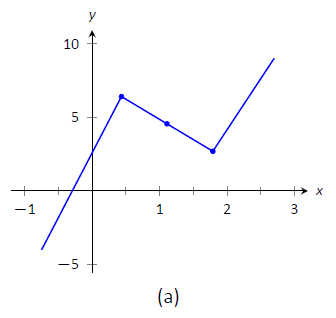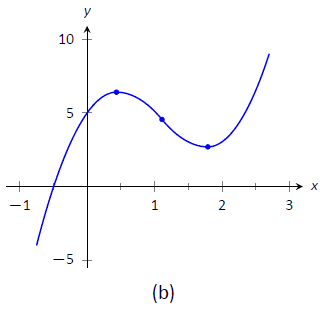Figure $$\PageIndex{2}$$: Sketching $$f$$ in Example $$\PageIndex{1}$$.

Example $$\PageIndex{2}$$: Curve sketching

Sketch $$f(x) = \dfrac{x^2-x-2}{x^2-x-6}$$.

Solution

We again follow the steps outlined in Key Idea 4.

1. In determining the domain, we assume it is all real numbers and looks for restrictions. We find that at $$x=-2$$ and $$x=3$$, $$f(x)$$ is not defined. So the domain of $$f$$ is $$D = \{\text{real numbers } x\ | \ x\neq -2,3\}$$.
2. To find the critical values of $$f$$, we first find $$f'(x)$$. Using the Quotient Rule, we find$$f'(x) = \frac{-8x+4}{(x^2+x-6)^2} = \frac{-8x+4}{(x-3)^2(x+2)^2}.$$$$f'(x) = 0$$ when $$x = 1/2$$, and $$f'$$ is undefined when $$x=-2,3$$. Since $$f'$$ is undefined only when $$f$$ is, these are not critical values. The only critical value is $$x=1/2$$.
3. To find the possible points of inflection, we find $$f''(x)$$, again employing the Quotient Rule:$$f''(x) = \frac{24x^2-24x+56}{(x-3)^3(x+2)^3}.$$We find that $$f''(x)$$ is never 0 (setting the numerator equal to 0 and solving for $$x$$, we find the only roots to this quadratic are imaginary) and $$f'$$is undefined when $$x=-2,3$$. Thus concavity will possibly only change at $$x=-2$$ and $$x=3$$.
4. The vertical asymptotes of $$f$$ are at $$x=-2$$ and $$x=3$$, the places where $$f$$ is undefined.
5. There is a horizontal asymptote of $$y=1$$, as $$\lim_{x\to -\infty}f(x) = 1$$ and $$\lim_{x\to\infty}f(x) =1$$.
6. We place the values $$x=1/2$$, $$x=-2$$ and $$x=3$$ on a number line as shown in Figure $$\PageIndex{3}$$. We mark in each interval whether $$f$$ is increasing or decreasing, concave up or down. We see that $$f$$ has a relative maximum at $$x=1/2$$; concavity changes only at the vertical asymptotes.FIgure $$\PageIndex{3}$$: Number line for $$f$$ in Example $$\PageIndex{2}$$

1. In Figure $$\PageIndex{4a}$$, we plot the points from the number line on a set of axes and connect the points with straight lines to get a general idea of what the function looks like (these lines effectively only convey increasing/decreasing information). In Figure $$\PageIndex{4b}$$, we adjust the graph with the appropriate concavity. We also show $$f$$ crossing the $$x$$ axis at $$x=-1$$ and $$x=2$$.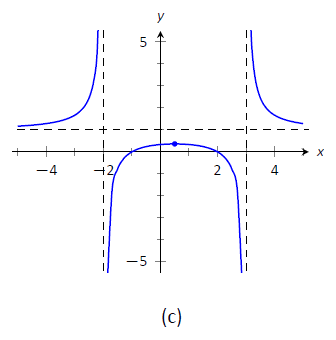Figure $$\PageIndex{4}$$: Sketching $$f$$ in Example $$\PageIndex{2}$$.

Figure $$\PageIndex{4c}$$ shows a computer generated graph of $$f$$, which verifies the accuracy of our sketch.

Example $$\PageIndex{3}$$: Curve sketching

Sketch $$f(x) = \frac{5(x-2)(x+1)}{x^2+2x+4}.$$

Solution

We again follow Key Idea 4

1. We assume that the domain of $$f$$ is all real numbers and consider restrictions. The only restrictions come when the denominator is 0, but this never occurs. Therefore the domain of $$f$$ is all real numbers, $$\mathbb{R}$$.
2. We find the critical values of $$f$$ by setting $$f'(x)=0$$ and solving for $$x$$. We find
$$f'(x) = \frac{15x(x+4)}{(x^2+2x+4)^2} \quad \Rightarrow \quad f'(x) = 0 \text{ when } \ x=-4,0.$$
3. We find the possible points of inflection by solving $$f''(x) = 0$$ for $$x$$. We find
$$f'p(x) = -\frac{30x^3+180x^2-240}{(x^2+2x+4)^3} .$$ The cubic in the numerator does not factor very "nicely." We instead approximate the roots at $$x= -5.759$$, $$x=-1.305$$ and $$x=1.064$$.
4. There are no vertical asymptotes.
5. We have a horizontal asymptote of $$y=5$$, as $$\lim_{x\to-\infty}f(x) = \lim_{x\to\infty}f(x) = 5$$.
6. We place the critical points and possible points on a number line as shown in Figure $$\PageIndex{5}$$ and mark each interval as increasing/decreasing, concave up/down appropriately.Figure $$\PageIndex{5}$$: Number line for $$f$$ in Example $$\PageIndex{3}$$.

1. In Figure $$\PageIndex{6a}$$ we plot the significant points from the number line as well as the two roots of $$f$$, $$x=-1$$ and $$x=2$$, and connect the points with straight lines to get a general impression about the graph. In Figure $$\PageIndex{6b}$$, we add concavity. Figure $$\PageIndex{6c}$$ shows a computer generated graph of $$f$$, affirming our results.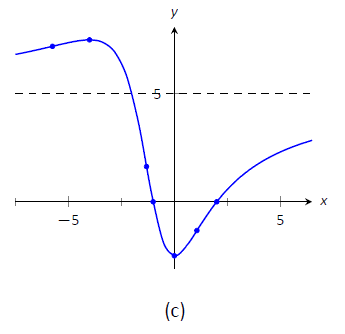Figure $$\PageIndex{6}$$: Sketching $$f$$ in Example $$\PageIndex{3}$$.

In each of our examples, we found a few, significant points on the graph of $$f$$ that corresponded to changes in increasing/decreasing or concavity. We connected these points with straight lines, then adjusted for concavity, and finished by showing a very accurate, computer generated graph.

Why are computer graphics so good? It is not because computers are "smarter" than we are. Rather, it is largely because computers are much faster at computing than we are. In general, computers graph functions much like most students do when first learning to draw graphs: they plot equally spaced points, then connect the dots using lines. By using lots of points, the connecting lines are short and the graph looks smooth.

This does a fine job of graphing in most cases (in fact, this is the method used for many graphs in this text). However, in regions where the graph is very "curvy," this can generate noticeable sharp edges on the graph unless a large number of points are used. High quality computer algebra systems, such as Mathematica, use special algorithms to plot lots of points only where the graph is "curvy.''

In Figure $$\PageIndex{7}$$, a graph of $$y=\sin x$$ is given, generated by Mathematica. The small points represent each of the places Mathematica sampled the function. Notice how at the "bends" of $$\sin x$$, lots of points are used; where $$\sin x$$ is relatively straight, fewer points are used. (Many points are also used at the endpoints to ensure the "end behavior" is accurate.)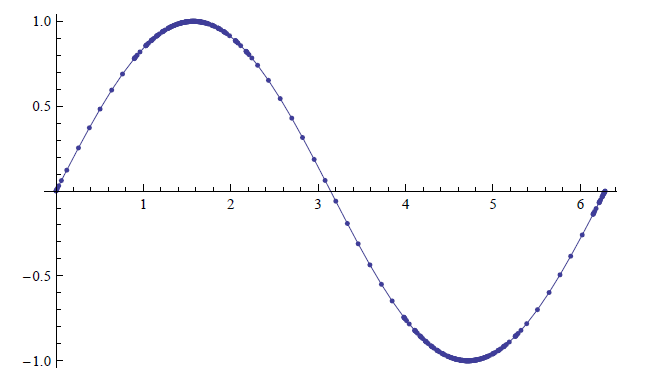Figure $$\PageIndex{7}$$: A graph of $$y=\sin x$$ generated by Mathematica.

How does Mathematica know where the graph is "curvy"? Calculus. When we study curvature in a later chapter, we will see how the first and second derivatives of a function work together to provide a measurement of "curviness." Mathematica employs algorithms to determine regions of "high curvature"' and plots extra points there.

Again, the goal of this section is not "How to graph a function when there is no computer to help.'' Rather, the goal is "Understand that the shape of the graph of a function is largely determined by understanding the behavior of the function at a few key places." In Example $$\PageIndex{3}$$, we were able to accurately sketch a complicated graph using only 5 points and knowledge of asymptotes!

There are many applications of our understanding of derivatives beyond curve sketching. The next chapter explores some of these applications, demonstrating just a few kinds of problems that can be solved with a basic knowledge of differentiation.

## Contributors

• Gregory Hartman (Virginia Military Institute). Contributions were made by Troy Siemers and Dimplekumar Chalishajar of VMI and Brian Heinold of Mount Saint Mary's University. This content is copyrighted by a Creative Commons Attribution - Noncommercial (BY-NC) License. http://www.apexcalculus.com/

• Integrated by Justin Marshall.